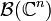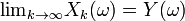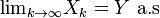# Almost sure convergence

Almost sure convergence is one of the four main modes of stochastic convergence. It may be viewed as a notion of convergence for random variables that is similar to, but not the same as, the notion of pointwise convergence for real functions.

## Definition

In this section, a formal definition of almost sure convergence will be given for complex vector-valued random variables, but it should be noted that a more general definition can also be given for random variables that take on values on more abstract topological spaces. To this end, let$(\Omega,\mathcal{F},P)$ be a probability space (in particular,$(\Omega,\mathcal{F}$) is a measurable space). A ($\mathbb{C}^n$-valued) random variable is defined to be any measurable function$X:(\Omega,\mathcal{F})\rightarrow (\mathbb{C}^n,\mathcal{B}(\mathbb{C}^n))$, where$\mathcal{B}(\mathbb{C}^n)$ is the sigma algebra of Borel sets of$\mathbb{C}^n$. A formal definition of almost sure convergence can be stated as follows:

A sequence$X_1,X_2,\ldots,X_n,\ldots$ of random variables is said to converge almost surely to a random variable Y if$\mathop{\lim}_{k \rightarrow \infty}X_k(\omega)=Y(\omega)$ for all$\omega \in \Lambda$, where$\Lambda \subset \Omega$ is some measurable set satisfying P(Λ) = 1. An equivalent definition is that the sequence$X_1,X_2,\ldots,X_n,\ldots$ converges almost surely to Y if$\mathop{\lim}_{k \rightarrow \infty}X_k(\omega)=Y(\omega)$ for all$\omega \in \Omega \backslash \Lambda'$, where Λ' is some measurable set with P(Λ') = 0. This convergence is often expressed as:$\mathop{\lim}_{k \rightarrow \infty} X_k = Y \,\,P{\rm -a.s},$

or$\mathop{\lim}_{k \rightarrow \infty} X_k = Y\,\,{\rm a.s}$.

## Important cases of almost sure convergence

If we flip a coin n times and record the percentage of times it comes up heads, the result will almost surely approach 50% as$n \rightarrow \infty$.

This is an example of the strong law of large numbers.Some content on this page may previously have appeared on Citizendium.